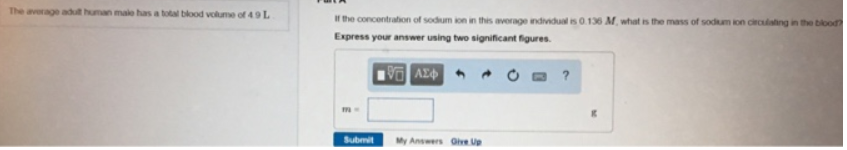# Problem: The average adult human male has a total blood volume of 4.9 L.If the concentration of sodium ion in this average individual is 0.136 M, what is the mass of sodium ion circulating in the blood? Express your answer using two significant figures.

###### FREE Expert Solution
81% (418 ratings)###### Problem Details

The average adult human male has a total blood volume of 4.9 L.

If the concentration of sodium ion in this average individual is 0.136 M, what is the mass of sodium ion circulating in the blood?